# Logic gates I

Digital Lab > Logic gates I

Inverter (NOT Gate) and Buffer

NAND and AND Gates

NOR and OR Gates

Digital Circuit operates at two different Voltage Levels, Low and High. Generally, the Low Level corresponds to Logic 0 and the High Level corresponds to Logic 1. Logic Gates are basic building blocks in Digital Electronics. The relation between the Input(s) and the Output of a Logic Gate is expressed with a Truth-Table.

### Inverter (NOT Gate) and Buffer

An Inverter is a Logic Gate that has only one Input, it outputs the opposite Logic State of its Input. The Inverter is also called NOT Gate. The Schematic Symbol of a basic Inverter is shown in Figure 1 and Table 1 is its Truth-Table.

The notation of the logic operation of Inverter can be expressed by: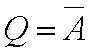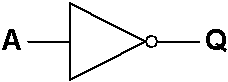Figure 1: Inverter (NOT Gate) Schematic Symbol

AQ
01
10

Table 1: Inverter (NOT Gate) Truth-Table

A Buffer is another Logic Gate that has only one Input, its Output follows the same Logic State as the Input. The Buffer is used as delay element in Digital Electronics. It is also a Current-Boost-Up element, which is used to increase the capability of the Output of one gate to drive a number of other gates. The Schematic Symbol of a Buffer is shown in Figure 2 and Table 2 is its Truth-Table.

The notation of the logic operation of Buffer can be expressed by: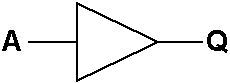Figure 2: Buffer Schematic Symbol

AQ
00
11

Table 2: Buffer Truth-Table

### NAND and AND Gates

An AND Gate is a Logic Gate that has two or more than two Inputs, its Output is 1 only when all its Inputs are 1. The Schematic Symbol of a Two-Input AND Gate is shown in Figure 3 and Table 3 is its Truth-Table.

The notation of the logic operation of AND Gate can be expressed by: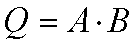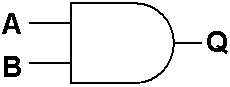Figure 3: AND Gate Schematic Symbol

ABQ
000
010
100
111

Table 3: AND Gate Truth-Table

A NAND Gate is another Logic Gate that has two or more than two Inputs, its Output is 0 only when all its Inputs are 1. The Schematic Symbol of a Two-Input NAND Gate is shown in Figure 4 and Table 4 is its Truth-Table.

The notation of the logic operation of NAND Gate can be expressed by: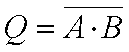Figure 4: NAND Gate Schematic Symbol

ABQ
001
011
101
110

Table 4: NAND Gate Truth-Table

### NOR and OR Gates

An OR Gate is a Logic Gate that has two or more than two Inputs, its Output is 0 only when all its Inputs are 0. The Schematic Symbol of a Two-Input OR Gate is shown in Figure 5 and Table 5 is its Truth-Table.

The notation of the logic operation of OR Gate can be expressed by: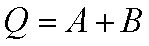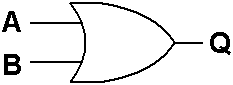Figure 5: OR Gate Schematic Symbol

ABQ
000
011
101
111

Table 5: OR Gate Truth-Table

A NOR Gate is a Logic Gate that has two or more than two Inputs, its Output is 1 only when all its Inputs are 0. The Schematic Symbol of a Two-Input NOR Gate is shown in Figure 6 and Table 6 is its Truth-Table.

The notation of the logic operation of NOR Gate can be expressed by: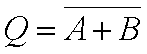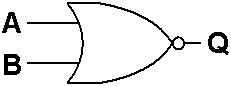Figure 6: NOR Gate Schematic Symbol

ABQ
001
010
100
110

Table 6: NOR Gate Truth-Table

Digital Lab > Logic gates I